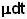## Saturday, June 7, 2008

### Irodov Problem 1.178The rate of change of momentum of the (rocket+fuel) system is equal to the sum of all the forces acting on the (rocket+ fuel) system.

Suppose that at some instant of time the mass of the rocket was . Suppose that over the next infinitesimally small interval of time dt, fuel of massshoots out if the rocket. The new mass of the rocket is thus now m-. Suppose the during this time the velocity of the rocket increases by dv, so its velocity is not v+dv. The velocity of the fuel relative to the rocket is u (this is a vector in the upward direction) relative to the rocket, so the velocity of the fuel relative to a stationary reference frame is v+u. The net change of momentum of the (rocket +fuel) system will be Fdt.

The change in momentum dP of the entire (rocket + fuel) system is given by,